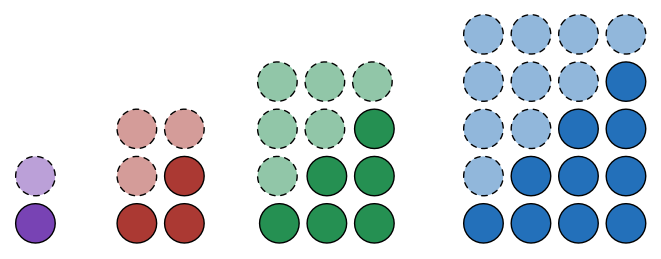# Triangular Numbers 4 – Find A Formula

Algebra Level 1Which of the following gives us a general formula for the size of the $n^{\text{th}}$ triangular number?

This problem is part of Arron's set What Makes A Number Triangular?

×